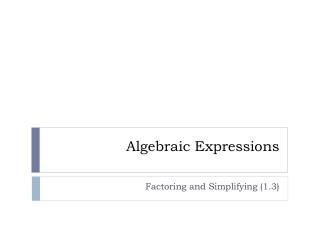DownloadDownload PresentationAlgebraic Expressions

# Algebraic Expressions

Download Presentation## Algebraic Expressions

- - - - - - - - - - - - - - - - - - - - - - - - - - - E N D - - - - - - - - - - - - - - - - - - - - - - - - - - -
##### Presentation Transcript

1. Algebraic Expressions Factoring and Simplifying (1.3)

2. POD– send answers via Navigator True or false? What’s your reasoning? (a ÷ b) ÷ c = a ÷ (b ÷ c)

3. SAT Question #1 Send your responses via Navigator. If then P = How did you get your answer?

4. SAT Question #2 Send your responses via Navigator. If find 6x. How did you get your answer?

5. SAT Question #3 Send your responses via Navigator. Simplify How did you get your answer?

6. Polynomials– The RULE: expressions with numbers and variables involving only addition, subtraction, and multiplication Following this rule, all exponents will be non-negative integers. We’re also assuming here that we combine all like terms. anxn + an-1xn-1+ an-2xn-2 + an-3xn-3 + … a1x + a0 Let’s start by reviewing the pieces. Some vocabulary: coefficient, leading coefficient variable degree monomial, binomial, trinomial linear, quadratic, cubic, etc. Is 5 a polynomial? Give an example of a polynomial and a non-polynomial.

7. Multiplying Multiply these: (2x + 3)(2x – 3) (2x + 3)2

8. Factoring– the opposite of multiplying What’s the first rule in factoring? Try these. You can verify your answers with CAS. x2 + x - 6 6x2 - 7x - 3 12x3 - 36x2y + 27xy2 a3 + b3 a3 - b3

9. Factoring– the opposite of multiplying What’s the first rule in factoring? Try these. You can verify your answers with CAS. x2 + x – 6 = (x + 3)(x – 2) 6x2 - 7x – 3 = (2x – 3)(3x + 1) 12x3 - 36x2y + 27xy2 = 3x(4x2 – 12xy + 9y2) = 3x(2x – 3)2 a3 + b3 = (a + b)(a2 – ab + b2) a3 - b3 = (a – b)(a2 + ab + b2)

10. Rational expressions • Let’s divide polynomials by other polynomials. • What are the restrictions on x? • Finish logistics…

11. Rational expressions Let’s divide polynomials by other polynomials. What are the restrictions on x? Finish logistics…